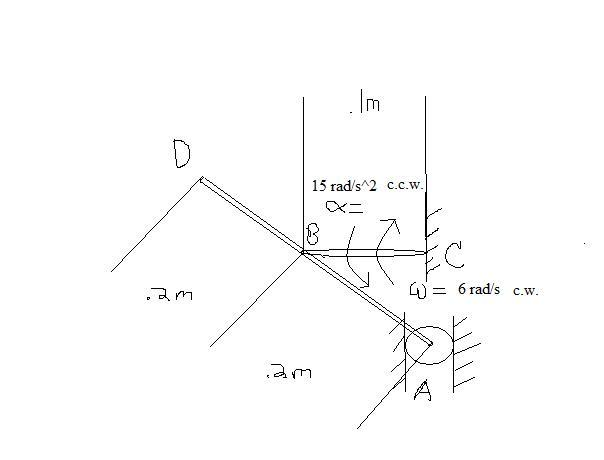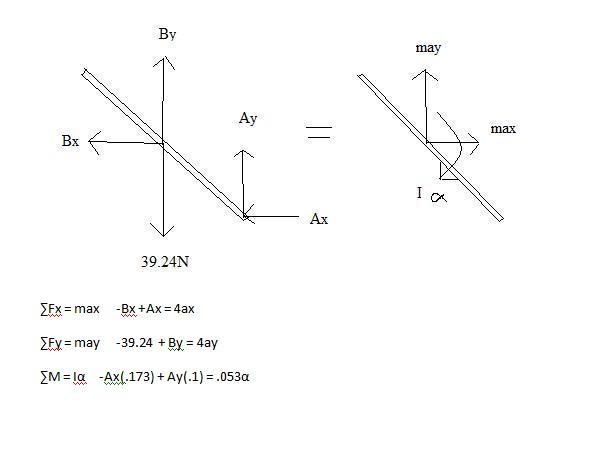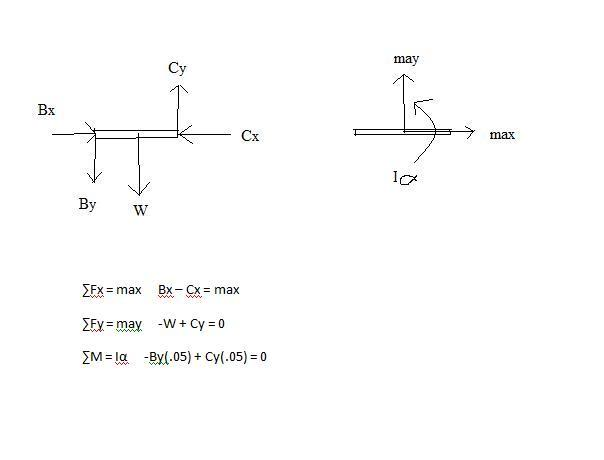# Multiple FBD Dynamics Problem

## Homework Statement

The 4-kg uniform rod ABD is attached to the crank BC and is fitted with a small wheel that can roll without friction along a vertical slot. Knowing that at the instant shown crank BC rotates with an angular velocity of 6 rad/s clockwise and an angular acceleration of 15 rad/s^2 counterclockwise, determine the reaction at A.## Homework Equations

Newton's 2nd Law (Shown in FBDs)
Acceleration and velocity kinematic equations
aa = ab + aa/b
va = vb + vc/b

## The Attempt at a Solution

I've drawn FBDs and written out Newton's 2nd Law, but I'm at a loss on how to write the kinematics equations I need. I know that they involve a cross product, but I don't really know how to use them to make an equation that will come out to all i's and j's. Please help!Thank you.